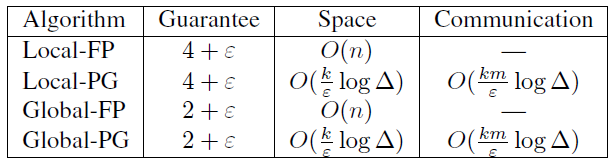# [ICDE07] Continuous Distributed Clustering

Author: Steven Date: May 22, 2022 Updated On: May 26, 2022
Categories: 经典聚类笔记
2k words in total, 8 minutes required.

Cormode, Graham, S. Muthukrishnan, and Wei Zhuang. “Conquering the Divide: Continuous Clustering of Distributed Data Streams.” In 2007 IEEE 23rd International Conference on Data Engineering, 1036–45, 2007. https://doi.org/10.1109/ICDE.2007.368962.

## 1. 聚类的概念

• k-median (minimizing the sum of distances from each point to its cluster center)

## 2. 集中式算法

### 2.1 Furthest Point Algorithm

$k$轮后，得到的结果针对于最优结果是2-factor approximation。

### 2.2 Parallel Guessing Algorithm

Parallel Guessing Algorithm提供$2+\epsilon$的approximation factor，其存储点数最多为$O(k/\epsilon \log \Delta)$，其中$\Delta$为集合中两点最远距离和最近距离的比值。

### 2.3 Merging Clusterings

$R_C \leq R_P$一直成立因为$C_i$的并是$P$的子集。

## 3. Continuous Distributed Clustering

### 3.1 Merging Local Solutions

#### 3.1.1 Local FP (Furthest Point)

site $i$ 以 $R_i$来维护聚类，当一个新点满足$d(C(p), p) \leq (1+\epsilon/2)R_i$，则最大的approximation为$2+\epsilon$，只需要把点合并到最近的$C(p)$。否则，需要reclustering，这时所有的点需要保存。每次reclustering会产生$O(k)$个点发送到centralized node。

#### 3.1.2 Local PG (Parallel Guessing)

site $i$ 以 最小的、生成不超过$k$个cluster的$R_i$来维护聚类。当$R_i$不再能满足新的到达点时，放大当前的$R_i$到$(1+\epsilon/2)$倍，并且和centralized进行通信。如果当前的guess少于$k$个centers并且出现了一个新的center，新的center会发送给centralized。同样，centralized node的approximation为$2+(2+\epsilon)$。

Site的存储为$O(k/\epsilon \log \Delta)$，则总共的通讯开销为$O(k m/\epsilon \log \Delta)$。

### 3.2 Maintaining Global Solutions

#### 3.2.1 Global FP

coordinator负责广播到各个site信息，并计算谁是广播的点的真正的最远的点，并把这个点再次广播，共进行$k+1$轮，直到选择出$k$个centers。

#### 3.2.2 Global PG

coordinator和remote site都保持multiple guesses。当一个remote site观测到一个新点，不能被某个$R$满足时，这个点被发送到coordinator。 coordinator判定这个点$p$是否可以被加入到remote site当前$R$中。

### 3.3 SummarySummary

• Global algorithms have better accuracy guarantees
• PG-based algorithms have smaller space requirements and communication guarantees

## 4. 扩展

### 4.3 1-center in Geometric Spaces

1-center即求一个minimum enclosing ball。但是上述随机选择第一个点的方法的approximation太大了。这里的特殊优化建议参照原文，稍显复杂。

## 扩展阅读

1. 1.T. Feder and D. H. Greene. Optimal algorithms for approximate clustering. In STOC, 1988.